Next: Spatial symmetry breaking Up: The chaotic pendulum Previous: Validation of numerical solutions

## The Poincaré section

For the sake of definiteness, let us fix the normalized amplitude and frequency of the external drive to be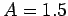and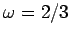, respectively.24 Furthermore, let us investigate any changes which may develop in the nature of the pendulum's time-asymptotic motion as the quality-factor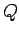is varied. Of course, ifis made sufficiently small (i.e., if the pendulum is embedded in a sufficiently viscous medium) then we expect the amplitude of the pendulum's time-asymptotic motion to become low enough that the linear analysis outlined in Sect. 4.2 remains valid. Indeed, we expect non-linear effects to manifest themselves asis gradually made larger, and the amplitude of the pendulum's motion consequently increases to such an extent that the small angle approximation breaks down.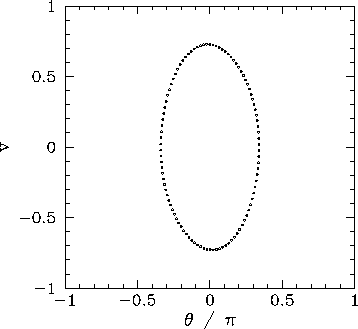Figure 28 shows a time-asymptotic orbit in phase-space calculated numerically for a case whereis sufficiently small (i.e.,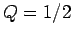) that the small angle approximation holds reasonably well. Not surprisingly, the orbit is very similar to the analytic orbits described in Sect. 4.2. The fact that the orbit consists of a single loop, and forms a closed curve in phase-space, strongly suggests that the corresponding motion is periodic with the same period as the external drive--we term this type of motion period-1 motion. More generally, period-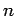motion consists of motion which repeats itself exactly everyperiods of the external drive (and, obviously, does not repeat itself on any time-scale less thanperiods). Of course, period-1 motion is the only allowed time-asymptotic motion in the small angle limit.

It would certainly be helpful to possess a graphical test for period-motion. In fact, such a test was developed more than a hundred years ago by the French mathematician Henry Poincaré--nowadays, it is called a Poincaré section in his honour. The idea of a Poincaré section, as applied to a periodically driven pendulum, is very simple. As before, we calculate the time-asymptotic motion of the pendulum, and visualize it as a series of points in-phase-space. However, we only plot one point per period of the external drive. To be more exact, we only plot a point when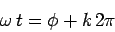(97)

whereis any integer, and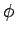is referred to as the Poincaré phase. For period-1 motion, in which the motion repeats itself exactly every period of the external drive, we expect the Poincaré section to consist of only one point in phase-space (i.e., we expect all of the points to plot on top of one another). Likewise, for period-2 motion, in which the motion repeats itself exactly every two periods of the external drive, we expect the Poincaré section to consist of two points in phase-space (i.e., we expect alternating points to plot on top of one another). Finally, for period-motion we expect the Poincaré section to consist ofpoints in phase-space.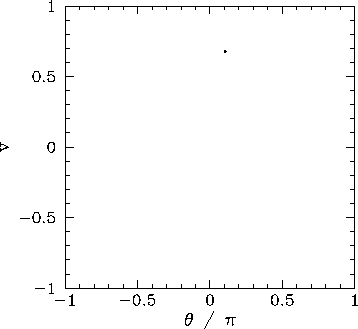Figure 29 displays the Poincaré section of the orbit shown in Fig. 28. The fact that the section consists of a single point confirms that the motion displayed in Fig. 28 is indeed period-1 motion.Next: Spatial symmetry breaking Up: The chaotic pendulum Previous: Validation of numerical solutions
Richard Fitzpatrick 2006-03-29